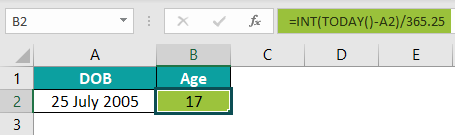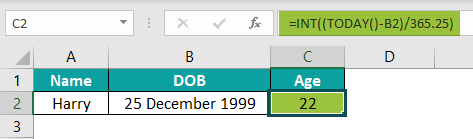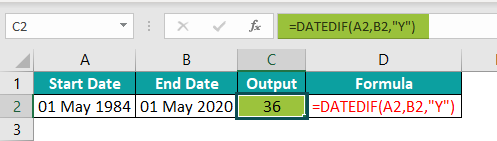# Calculate Age In ExcelArticle byExcelMojo Team## Calculate Age In Excel

We use the Calculate Age in Excel feature to find a person’s age with the help of their date of Birth. We can also use the TODAY and DATEDIF functions. Excel does not have a pre-defined formula for the same. Therefore, we can use the following Basic Formula to Calculate Age in Excel, =(Today’s Date – Date of Birth) / 365

###### Key Takeaways
• The basic formula to Calculate Age in Excel is (Today’s Date – Date of Birth) / 365. So we need the Date of Birth and Today’s date.
• We can use a few other functions such as the DATEDIF(), YEARFRAC(), and TODAY() to Calculate Age in Excel.
• The two dates compared to Calculate Age in Excel must be in the same format. Therefore, if we get the results or output in text format, general, or any other format, convert them to the date or number option using the “Format Cells” window of Excel.

### Top 3 Methods To Calculate Age In Excel

We will see the top three ways to Calculate Age In Excel, namely,

1. Basic Excel Formula for Age in Years.
2. Calculate Age using the YEARFRAC function.
3. Calculate Age using the DATEDIF function.

#### #1 – Basic Excel Formula for Age in Years

We will use the Basic Formula to Calculate Age in Excel to find the age in years.

In the following table, the data is,

• Column A shows the Name.
• Column B contains the Date of Birth.
• Column C for the Output [Age].

The steps to Calculate Age from Date of Birth in Excel using the basic formula are as follows:

Step 1: Select cell C2 and enter the formula =(TODAY()-B2)/365.

Step 2: Press the “Enter” key. The output is ‘27.655030801’, as shown below.

Step 3: As the output is a decimal, not an integer, we will enter the INT formula. Then, the complete formula will be =INT((TODAY()-B2)/365.25).

Step 7: Press the “Enter” key. Now, the exact age is ‘27’.

#### #2 – Calculate Age using the YEARFRAC function

We will first understand the YEARFRAC function.

YEARFRAC function – It calculates the number of whole days between two dates.

The syntax of the YEARFRAC function is,

The arguments of the YEARFRAC function are,

• start_date – It denotes the start date. It is a mandatory argument.
• end_date – It denotes the end date. It is a mandatory argument.
• basic – It denotes dates fraction. It is an optional argument.

We will use the YEARFRAC function to Calculate Age from Date of Birth in Excel.

In the following table, the data is,

• Column A shows the Name.
• Column B contains the Date of Birth.
• Column C for the Output [Age].

The steps to Calculate Age in Excel using the YEARFRAC function are as follows:

Step 1: Select cell C2 and enter the =YEARFRAC(B2,TODAY(),1).

[Note: The start_date, i.e., the DOB, is in cell B2. We will take the end_date as today’s date, so use the Today() function and the basic is 1].

Step 2: Press the “Enter” key. The output is ‘31’, as shown in the image below.

#### #3 – Calculate Age using the DATEDIF function

We will first understand the DATEDIF function.

DATEDIF function – It calculates the number of whole days, full months, or full years between two dates.

The syntax of the DATEDIF function is,

=DATEDIF(start_date,end_date,unit)

The arguments of the DATEDIF function are,

• start_date – It is the start date of the period we want to calculate. It is a mandatory argument.
• end_date – It is the end date of the period we want to calculate. It is a mandatory argument.
• unit – It is the time unit we want to calculate. It is a mandatory argument.

The Unit table is as follows:

We will use the DATEDIF function to Calculate Age in Excel.

In the following table, the data is,

• Column A shows the Start Date.
• Column B contains the End Date.
• Column C for the Output [Age].

The steps to Calculate Age in Excel using the DATEDIF function are as follows:

Step 1: Select the column C and add the formulas along with their units as follows,

• In cell C2, enter the formula =DATEDIF(A2,B2,”Y”)
• In cell C3, enter the formula =DATEDIF(A3,B3,”M”)
• In cell C4, enter the formula =DATEDIF(A4,B4,”D”)
• In cell C5, enter the formula =DATEDIF(A5,B5,”MD”)
• In cell C6, enter the formula =DATEDIF(A6,B6,”YD”)
• In cell C7, enter the formula =DATEDIF(A7,B7,”YM”)

Step 2: After entering the formulas in their respective cells, press the “Enter” key.

We will get the above output shown in Column C. [Note: The results are as per the specified units, w.r.t days, months, years, etc. Column D is for our reference of the applied formulas].

### Other Examples Of Age Calculation

We will consider a few other methods to Calculate Age in Excel, namely,

1. DATEDIF and Arithmetic Operations to Calculate Age in Completed and Fractional Months.
2. CONCATENATE and DATEDIF to Calculate Age in Year, Months, and Days.

#### #1 – DATEDIF and Arithmetic Operations to Calculate Age in Completed and Fractional Months

We will Calculate Age in Excel using the DATEDIF and Arithmetic Operations in Completed and Fractional Months.

In the following table, the data is,

• Column A shows the Start date.
• Column B contains the End Date.
• Column C is for output [Age].

The steps to Calculate Age in Excel, w.r.t months, are as follows:

Step 1: Select cell C2 and enter the formula =DATEDIF(A2, B2, “M”)

Step 2: Press the “Enter” key. The output is ‘340’ months, as shown below.

Step 3: Drag the formula from cell C7 to C8 using the fill handle.

We will get the output as shown above i.e., the number of months between the start and end dates.

#### #2 – CONCATENATE and DATEDIF to Calculate Age in Year, Months, and Days

We will Calculate Age in Excel using the CONCATENATE function and DATEDIF in Years, Months, and Days.

In the following table, the data is,

• Column A shows the Start date.
• Column B contains the End Date.
• Column C is for output [Age].

The steps to Calculate Age in Excel, w.r.t days, months, and years, are as follows:

Step 1: Enter the complete formula =CONCATENATE(DATEDIF(A2,B2,”Y”),”Years “,DATEDIF(A2,B2,”YM”),”Months and “,DATEDIF(A2,B2,”MD”),”Days”) in cell C2, as shown below.

Step 2: Press the “Enter” key. The result is ‘28Years 1Months and 9days’ in the image below.

Step 3: Drag the formula from cell C2 to C7 using the fill handle.

We will get the output shown above. One formula gives the total years, months, and days.

### Important Things To Note

• If we copy the DATEDIF function to another cell in Excel, we will get the #NUM! error or just 0 in the cell. Therefore, we should enter the formula manually or drag it into a dataset since it is not an inbuilt function in Excel.
• We will get the #NUM! error if the end date is less than the start date.
• If Excel does not recognize the valid dates, it returns the “#VALUE!” error.

What is the Formula to Calculate Age in Excel?

The Formula to Calculate Age in Excel is,

(Today’s Date – Date of Birth) / 365

We can use 365.25 for counting a leap year which appears every four years.

For example, the DOB is “25 July 2005”, and Today’s date is “20 June 2022”, divided by 365.25.

The complete formula is =INT(TODAY()-A2)/365.25. The result is ‘17’.How to Calculate Age from Date of Birth in Excel?

In the following image, the example shows the Date of Birth of Mr. Harry, and we will Calculate Age from Date of Birth in Excel.

The DOB is “25 December 1999,” and Today’s date is “20 June 2022”, divided by 365.25.

We can use 365.25 for counting a leap year which appears every four years. We use the INT function to get integer values as output, not decimals.

The complete formula is =INT(TODAY()-B2)/365.25. The result is ‘22’.How to Calculate Age in Excel between two dates?

In the following image, the example shows a person’s Start Date and End Date, and we will Calculate Age in Excel between these two dates.

The complete formula is =DATEDIF(A2, B2, “Y”). The result is ‘36’.